# Get parts along curved path formed by Point A and Point B

Hello there. I will try to make this as short as possible. I want to be able to get any part along a path that is generated via Point A and Point B. I can easily do that using a straight path, but I have no idea how to do this using a curved path. I tried researching Bezier Curves, but I simply cannot understand them.

I am going to most likely be using this for a train path system, so I do want the curve to be smooth and look natural (in a way that curves of such type would look like in a real track). Here is a rough example I drew in paint:

Example

In short, I have Part A and Part B, both at different orientations (with their front-face pointing at the direction of the track), and I want to be able to generate a curved path (or at least get the CFrames of that curved path). I would assume Bezier Curves would be the way to go, but they require three points instead of two. Is there possibly another way I could approach this problem without a Bezier Curve? If not, could someone give me an example or lead me to some tutorials that would help me understand Bezier Curves and how to approach this problem?

That is all. Thank you for your time and help, I greatly appreciate it.

you could try using pathfindingservice but that probably isnt the best thing to rely on

1 Like

Thank you for the reply. I do not think PathFindingService would work with this. If I am correct, PathFindingService finds the shortest path from Point A to Point B, which would definitely not be a smooth curve, and most likely not even a curve either. I did think of using TweenService or Lerp, but it is not going to have the curving behavior, it is just going to move in a straight line towards from Point A to Point B, and just change the rotation on the way. Thank you for the reply though.

1 Like

1 Like

In order to get the result you desire, you’ll need more than simply a point A and a point B

This should help simplify things on your end

1 Like

you could do it like this

in a loop do

place a part like a tiny distance in front of part A

then Place a Part in front of that part using look Vectors. but rotate it by a fraction of the rotation of Part B.

then if you continuously keep doing this there will eventually be a part on part B with the same position,Lookvector,orientation

May sound confusing but i think it’ll work, id code it myself but I’m not to familiar with lookVectors lol

then just

1 Like

Thank you all for the replies. After some YouTube, some more researching, and a little bit of thinking, I was able to figure out exactly what I need to get this working. Yes, I did indeed need to use Bezier Curves, but the issue was where Point B would be located to make it smooth.

To make this short, Point B must be in the “hypothetical” middle of the Point A and Point B if Point B was directly in front of Point A.

Visual Representations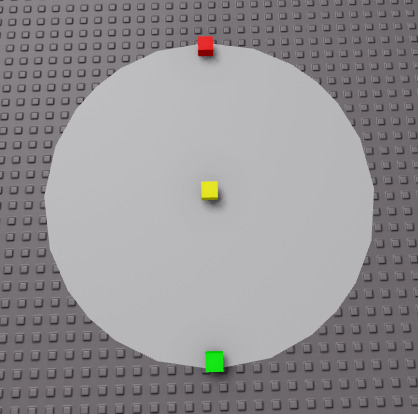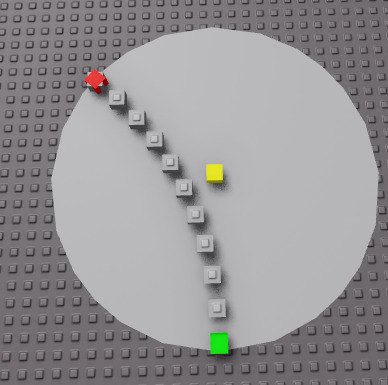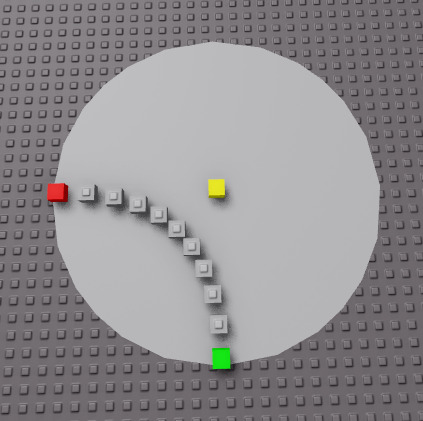In order to get Point B when you have Point A and Point C, I would assume the best way to do so is creating two lines based on the LookVector and then finding the intersecting point. (I have not figured out how to do this yet, but since I can ignore the Y axis, I basically have a 2D point, and I just need to get the slope of those lines in order to find the intersection)

Example

Intersection point: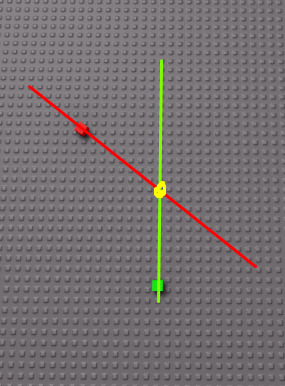Actual point: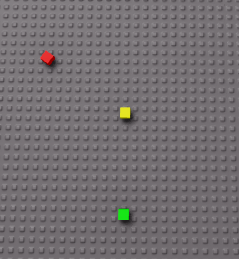I hope this helps any future developer who encounters the same question or problem as me. Cheers!

1 Like